NCERT Solutions: Pair of Linear Equations in Two Variables (Exercise 3.1)

# Pair of Linear Equations in Two Variables (Exercise 3.1) NCERT Solutions - Mathematics (Maths) Class 10

Q1. Aftab tells his daughter, “Seven years ago, I was seven times as old as you were then. Also, three years from now, I shall be three times as old as you will be.” (Isn’t this interesting?) Represent this situation algebraically and graphically.

Let the present age of Aftab be ‘x’.
And, the present age of his daughter be ‘y’.
Now, we can write, seven years ago,

• Age of Aftab = x - 7
• Age of his daughter = y - 7

According to the question, x − 7 = 7(y − 7)
⇒ x − 7 = 7y − 49
⇒ x − 7y = −42 ………………………(i)
Also, three years from now or after three years:

• Age of Aftab will become = x + 3.
• Age of his daughter will become = y + 3

According to the situation given:
x + 3 = 3(y + 3)
⇒ x + 3 = 3y + 9
⇒ x − 3y = 6 …………..…………………(ii)
Subtracting equation (i) from equation (ii) we have:
(x − 3y) − (x − 7y) = 6 − (−42)
⇒ −3y + 7y = 6 + 42
⇒ 4y = 48
⇒ y = 12
The algebraic equation is represented by:
x − 7y = −42
x − 3y = 6
For, x − 7y = −42 or x = −42 + 7y
The solution table is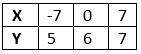For,  x − 3y = 6 or x = 6 + 3y
The solution table is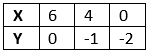The graphical representation is: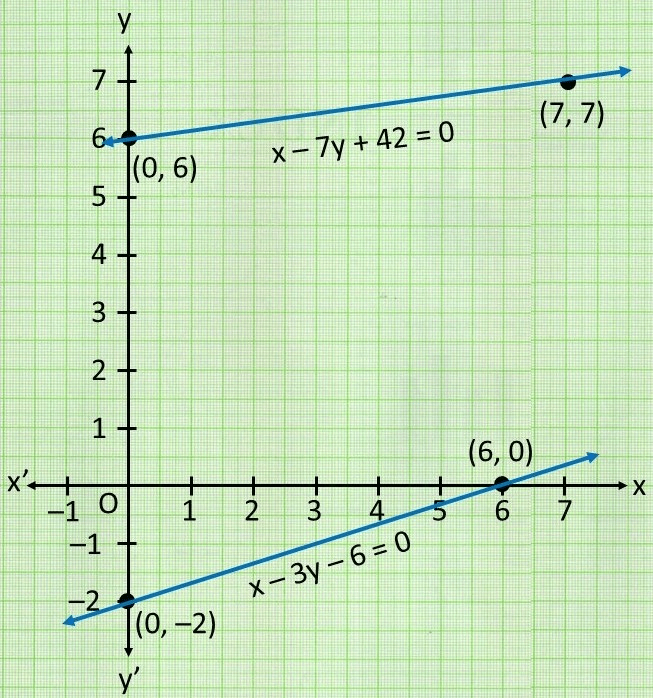Q2. The coach of a cricket team buys 3 bats and 6 balls for Rs.3900. Later, she buys another bat and 3 more balls of the same kind for Rs.1300. Represent this situation algebraically and geometrically.

Let us assume that the cost of a bat be ‘Rs x’
And, the cost of a ball be ‘Rs y’
According to the question, the algebraic representation is:
3x + 6y = 3900
And x + 3y = 1300
For, 3x + 6y = 3900
Or x = (3900 - 6y) / 3
The solution table is: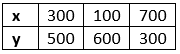For, x + 3y = 1300
Or x = 1300 - 3y
The solution table is: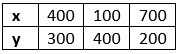The graphical representation is as follows: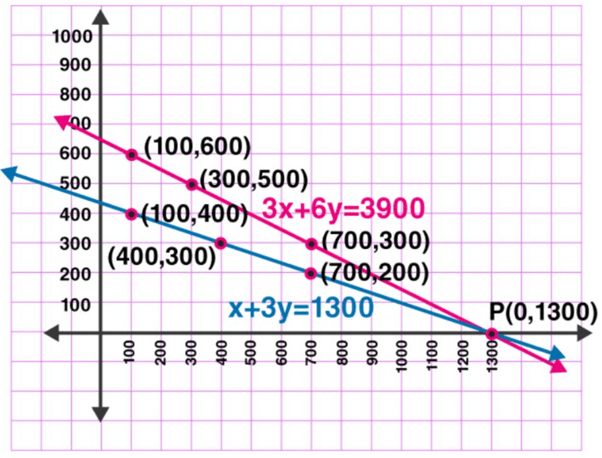Q3. The cost of 2 kg of apples and 1kg of grapes on a day was found to be Rs.160. After a month, the cost of 4 kg of apples and 2 kg of grapes is Rs.300. Represent the situation algebraically and geometrically.

Let the cost of 1 kg of apples be ‘Rs. x’
And, cost of 1 kg of grapes be ‘Rs. y’
According to the question, the algebraic representation is:
2x + y = 160 And 4x + 2y = 300
For, 2x + y = 160 or y = 160 − 2x, the solution table is: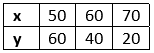For 4x + 2y = 300 or y = (300 - 4x) / 2, the solution table is: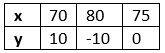The graphical representation is as follows: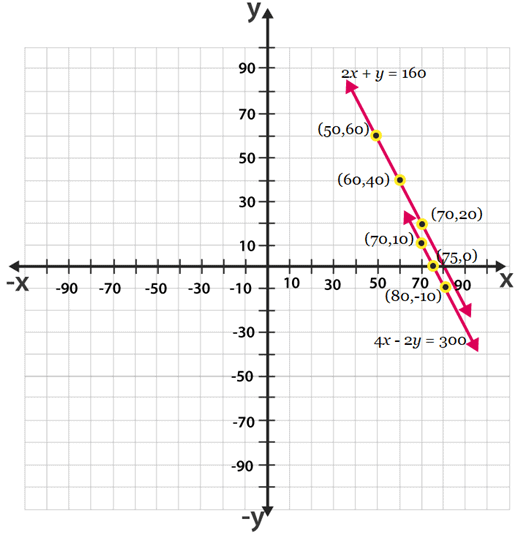Check out the NCERT Solutions of all the exercises of Linear Equations in Two Variables:

The document Pair of Linear Equations in Two Variables (Exercise 3.1) NCERT Solutions | Mathematics (Maths) Class 10 is a part of the Class 10 Course Mathematics (Maths) Class 10.
All you need of Class 10 at this link: Class 10

## Mathematics (Maths) Class 10

115 videos|478 docs|129 tests

## FAQs on Pair of Linear Equations in Two Variables (Exercise 3.1) NCERT Solutions - Mathematics (Maths) Class 10

 1. What are linear equations in two variables?Ans. Linear equations in two variables are algebraic expressions that involve two variables and have a degree of one. The general form of a linear equation in two variables is ax + by + c = 0, where a, b, and c are constants and x and y are variables.
 2. How do we solve a pair of linear equations in two variables?Ans. To solve a pair of linear equations in two variables, we need to find the values of x and y that satisfy both equations simultaneously. There are different methods to solve such equations, such as substitution method, elimination method, and graphical method.
 3. What is the importance of linear equations in two variables in real-life applications?Ans. Linear equations in two variables have a wide range of applications in real-life situations, such as in economics, physics, engineering, and business. For example, they can be used to model linear relationships between two variables, such as the relationship between the demand and supply of a product, the distance and time traveled by a vehicle, or the cost and revenue of a business.
 4. Can a pair of linear equations in two variables have more than one solution?Ans. Yes, a pair of linear equations in two variables can have more than one solution, no solution, or a unique solution, depending on the nature of the equations. For example, if the two equations are parallel, they have no solution. If they are identical, they have infinitely many solutions. If they intersect at a single point, they have a unique solution.
 5. How does the graphical method of solving a pair of linear equations work?Ans. The graphical method of solving a pair of linear equations involves plotting the two equations on the same coordinate plane and finding the point of intersection, if any. The point of intersection represents the values of x and y that satisfy both equations simultaneously. If the lines are parallel, they do not intersect and have no solution. If they coincide, they have infinitely many solutions.

## Mathematics (Maths) Class 10

115 videos|478 docs|129 testsExplore Courses for Class 10 examSignup to see your scores go up within 7 days! Learn & Practice with 1000+ FREE Notes, Videos & Tests.
10M+ students study on EduRev
Track your progress, build streaks, highlight & save important lessons and more!
Related Searches

,

,

,

,

,

,

,

,

,

,

,

,

,

,

,

,

,

,

,

,

,

;